Polar representation

Chapter 5 Class 11 Complex Numbers
Concept wise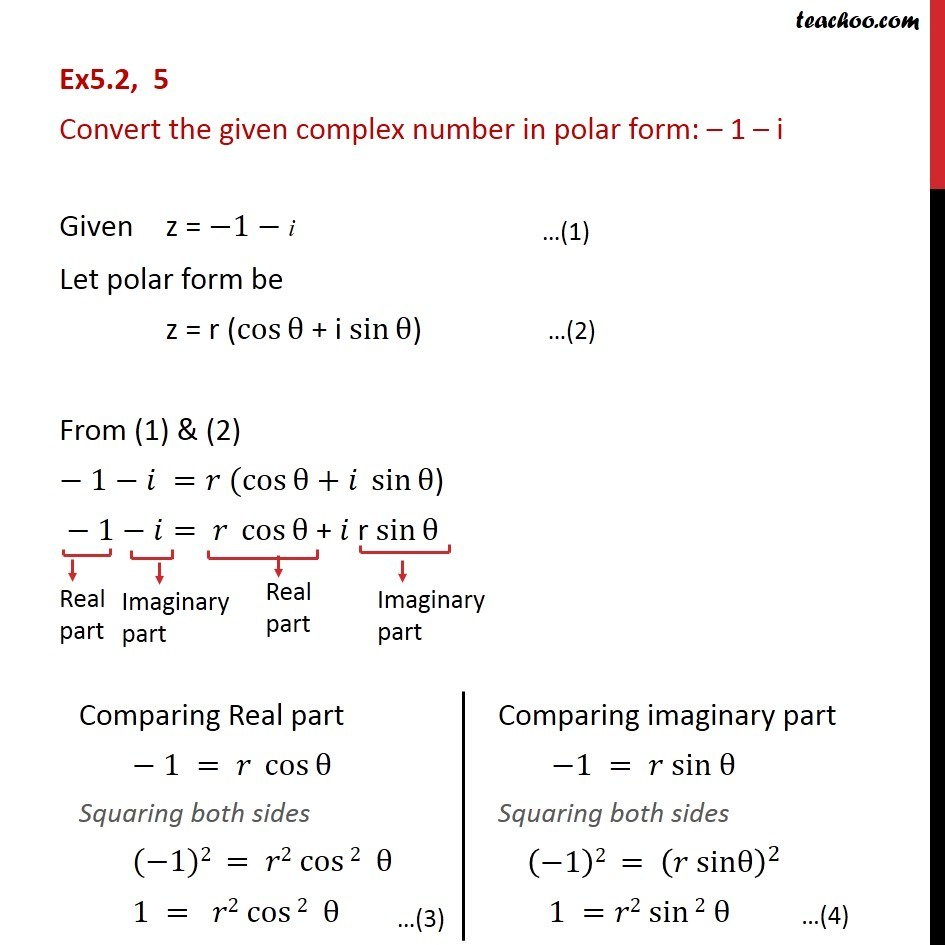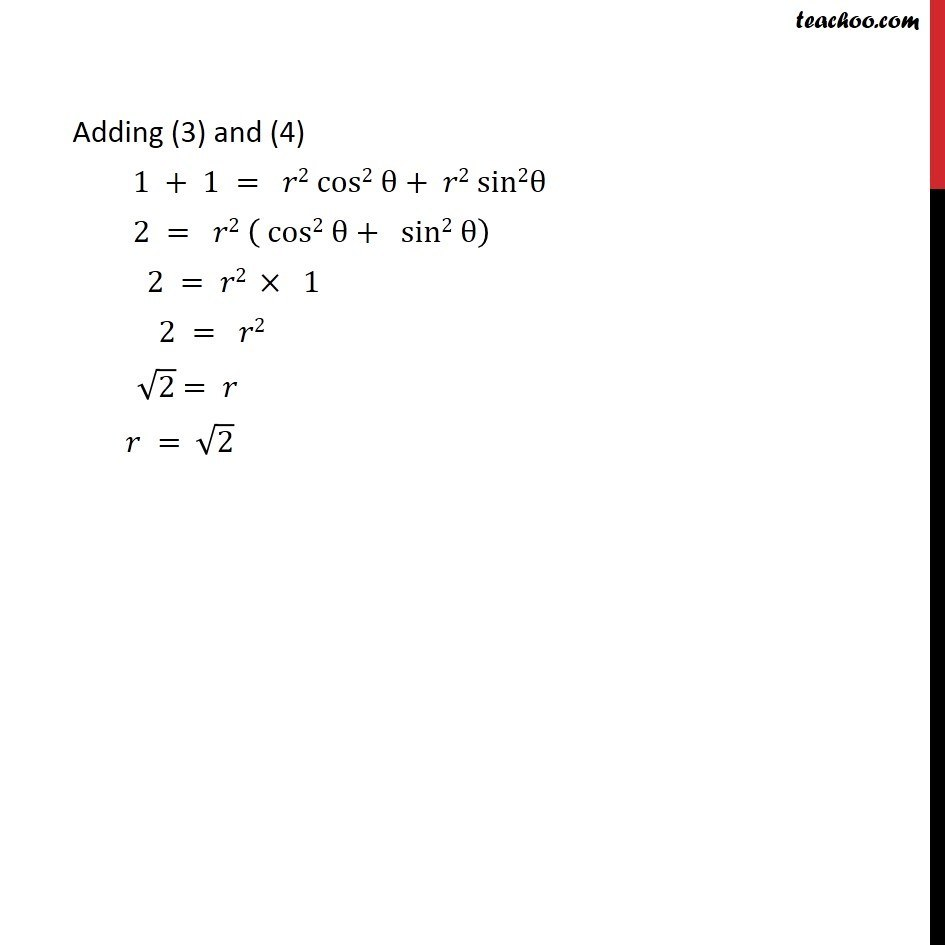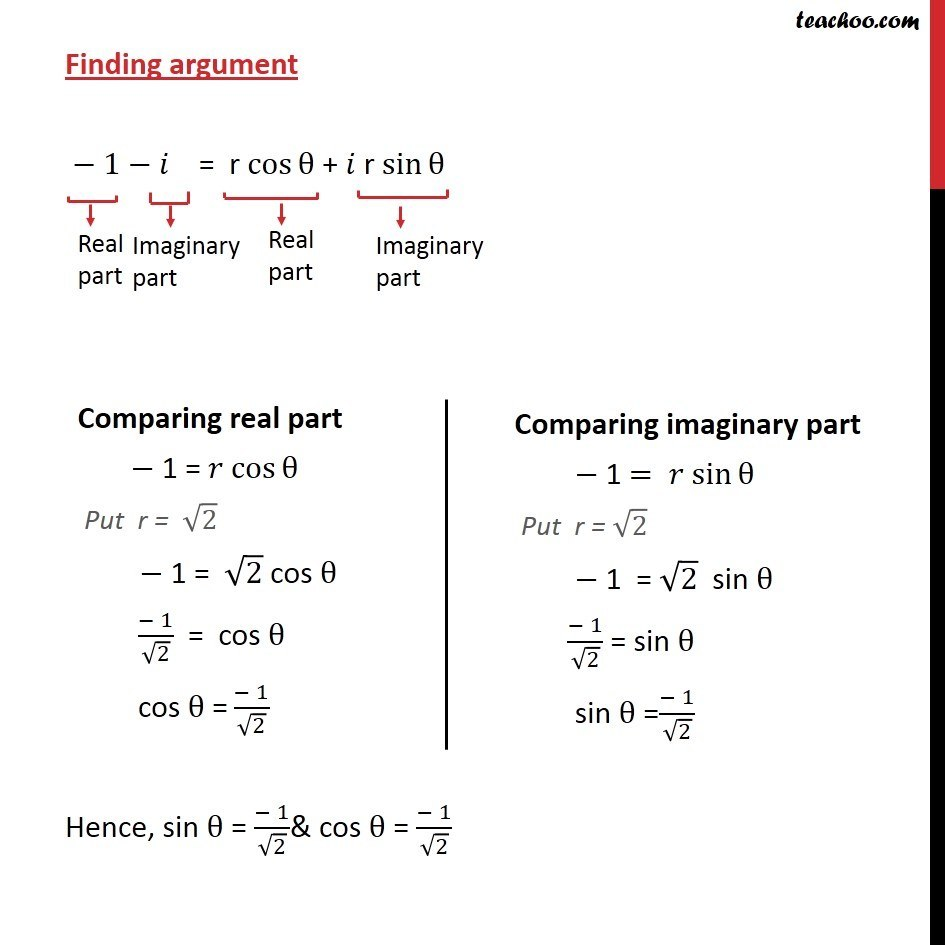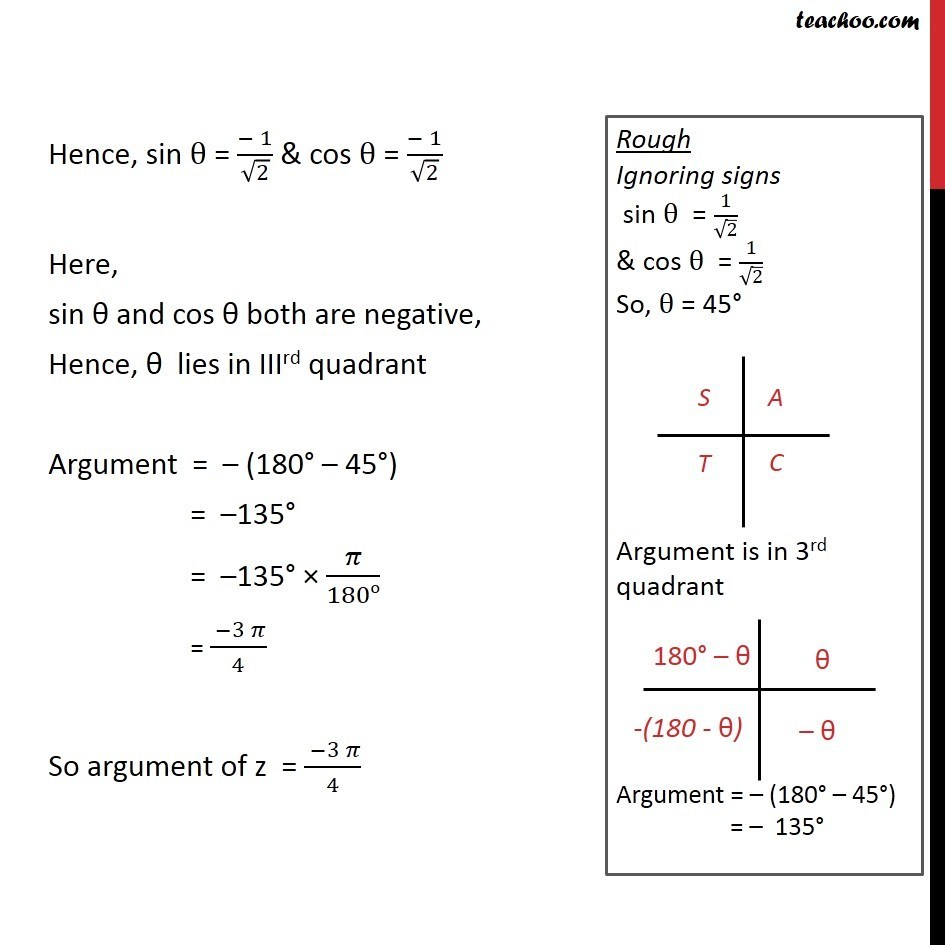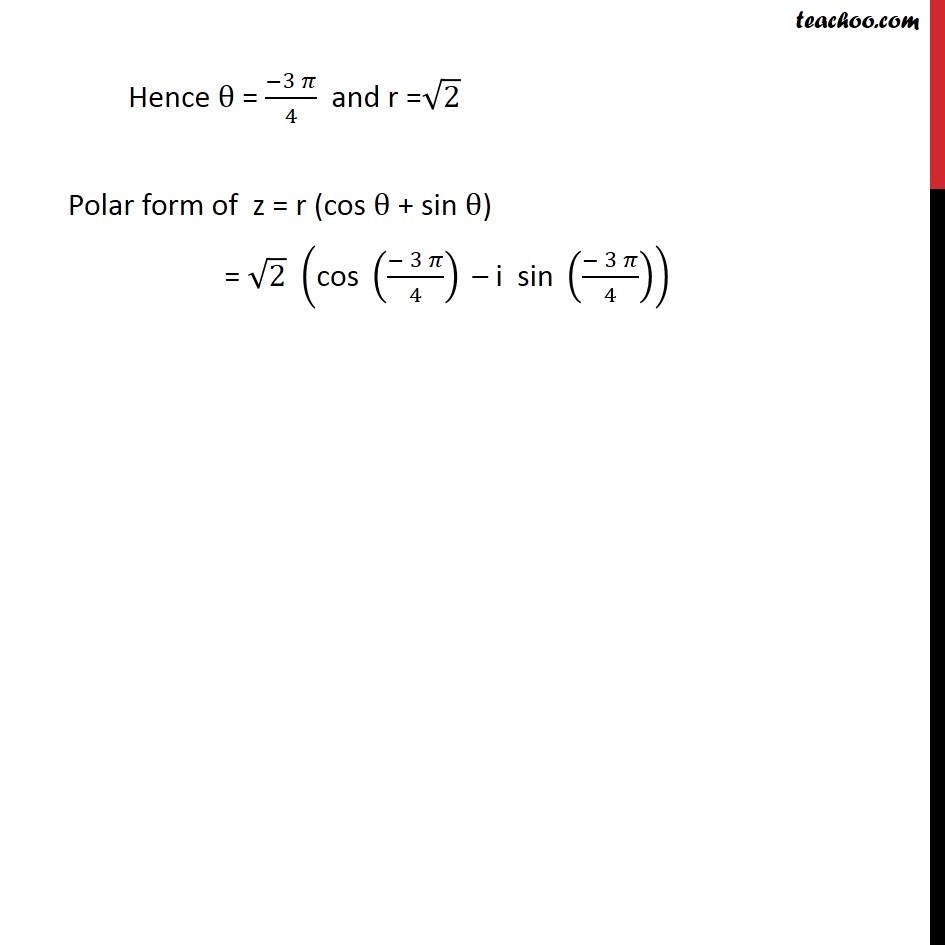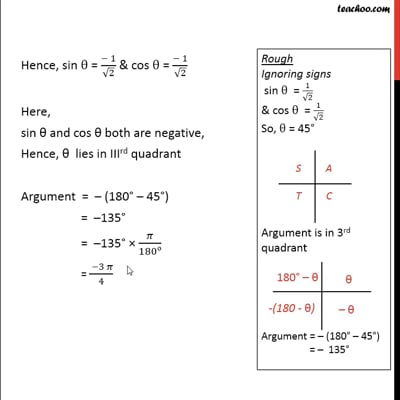This video is only available for Teachoo black users

Introducing your new favourite teacher - Teachoo Black, at only ₹83 per month

### Transcript

Ex5.2, 5 Convert the given complex number in polar form: – 1 – i Given z = −1− i Let polar form be z = r (cos⁡θ + i sin⁡θ) From (1) & (2) − 1−𝑖 =𝑟 (cos⁡θ+𝑖 sin⁡θ) − 1−𝑖= 𝑟 〖 cos〗⁡θ + 𝑖 r sin⁡θ Adding (3) and (4) 1 + 1 = 𝑟2 cos2 θ+ 𝑟2 sin2θ 2 = 𝑟2 ( cos2 θ+ sin2 θ) 2 = 𝑟2 × 1 2 = 𝑟2 √2 = 𝑟 𝑟 = √2 Finding argument − 1− 𝑖 = r〖 cos〗⁡θ + 𝑖 r sin⁡θ Hence, sin θ = (− 1)/√2& cos θ = (− 1)/√2 Hence, sin θ = (− 1)/√2 & cos θ = (− 1)/√2 Here, sin θ and cos θ both are negative, Hence, θ lies in IIIrd quadrant Argument = – (180° – 45°) = –135° = –135° × 𝜋/180o = ( −3 𝜋)/4 So argument of z = ( −3 𝜋)/4 Hence θ = (−3 𝜋)/4 and r =√2 Polar form of z = r (cos θ + sin θ) = √2 ("cos " ((− 3 𝜋)/4)" – i sin " ((− 3 𝜋)/4))